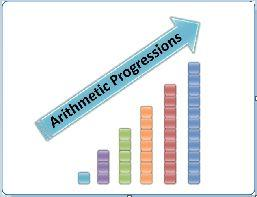# Facts that Matter- Arithmetic Progressions Class 10 Notes | EduRev

## Class 10 : Facts that Matter- Arithmetic Progressions Class 10 Notes | EduRev

The document Facts that Matter- Arithmetic Progressions Class 10 Notes | EduRev is a part of the Class 10 Course Class 10 Mathematics by VP Classes.
All you need of Class 10 at this link: Class 10

Facts that Matter

• Sequence:
When some numbers are arranged in a definite order, according to a definite rule, they are said to form a sequence.
The number occurring at the 1st place is called the 1st term, denoted by T1.
The number occurring at the nth place is called the nth term, denoted by Tn.
Example: Let us consider a rule
Tn = 3n + 1
Putting n = 1, 2, 3, 4, 5, ..... we get
T1 = 3 (1) + 1 = 4
T2 = 3 (2) + 1 = 7
T3 = 3 (3) + 1 = 10
T4 = 3 (4) + 1 = 13
T5 = 3 (5) + 1 = 16
......    ......    ......
......    ......    ......
......    ......      ......
∴ The numbers 4, 7, 10, 13, 16, ..... ..... form a sequence.
The pattern followed by its terms is:
• Finite Sequence
A sequence containing definite number of terms is called a finite sequence.
• Infinite Sequence
A sequence containing infinite number of terms is called an infinite sequence.
• ProgressionsThe sequences in which each term (other than the first and the last) is related to its succeeding term by a fixed rule, are called progressions.

Note:
There are three types of progressions:
I. Arithmetic Progressions (A.P.)
II. Geometric Progressions (G.P.)
III. Harmonic Progressions (H.P.)
But, here we shall learn about arithmetic progression.

• Arithmetic Progression (A.P.)
An arithmetic progression is a list of numbers in which each term is obtained by adding a fixed number to the preceding term except the first term.
The fixed number is called the common difference, which can be positive, negative or zero.
The common difference of an A.P. can be obtained by subtracting any term from its following term.
Generally the first term is denoted by ‘a’ and the common difference is denoted by ‘d’.
• If three numbers a, b, c are in order, then (b – a) = common difference = (c – d)
•  When certain number of terms of an A.P. are required, then we select the terms in the following manner:
Number of Terms, Terms and Common difference: Number of Terms Terms Common difference 3 a – d, a, a + d d 4 a – 3d, a – d, a + d, a + 3d 2d 5 a – 2d, a – d, a, a + d, a + 2d d 6 a – 5d, a – 3d, a – d, a + d, a + 3d, a + 5d 2d
Offer running on EduRev: Apply code STAYHOME200 to get INR 200 off on our premium plan EduRev Infinity!

132 docs

,

,

,

,

,

,

,

,

,

,

,

,

,

,

,

,

,

,

,

,

,

;